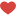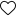In this blog I cover some basic definitions of different terms used in Statistics and then we will jump to the most powerful theorem in statistics – “The Central Limit Theorem”

Population : A population consists of the entire collection of observations which we are concerned about.

Sample : A sample is a subset of the population

Sampling : A method to select sample(s) from population

Simple Random Sampling : A method to select sample elements from population, in which all sample observations are taken at random and assume independence.

Parameter : A measurable characteristic of a population, such as a mean or standard deviation, is called a parameter

Statistic : A measurable characteristic of a sample is called a statistic

Experiment: An experiment is a set of positive outcomes that can be repeated

Sample Space : A set of all possible outcomes of an experiment is a Sample Space.

Example : Tossing a coin is an experiment, list of its possible outcomes i.e {Head, Tail}

is sample space.

Event and Event Space : An event is a positive outcome in which we are interested.

For eg. tossing a coin twice , possible outcomes are {HH, HT, TH, TT}, these form sample space.

Say we are interested in event in which we get at least one H.

So positive outcomes are {HH, HT, TH} which form event space

Random Variable : A random variable, denoted by X, is a function that associates

a real number with every outcome of an experiment.

Discrete Random Variable : We say X is a discrete random variable if it can assume at most a finite or a countably infinite number of possible values. If we trow a dice we can get a number between 1 and 6
Sampling distribution of Sample Means:

Lets say we measure height of Women playing Basketball at National level. This forms population. Now from this population we randomly select a sample of size 30 and find mean height of Women in that sample. Similarly we draw another sample of same size (30) and calculate mean height of women in second sample, and we repeat this process many times. Every time we calculate a sample mean we add it to a set and this set of sample means is called as sampling distribution or to be more specific sampling distribution of sample means.

Central Limit Theorem (CLT):

CLT says that sampling distribution of sample means follow normal distribution. There is a very surprising feature concerning the central limit theorem. The astonishing fact as per CLT is that – a normal distribution arises regardless of the underlying distribution of population.

As per CLT, mean of sample means is equal to population mean .
and standard deviation of sample means = population standard deviation / Sqrt(n)
where n in sample size.

Application of CLT

Lets say an elevator has max capacity to carry load is 1050 Kg and atmost 12 people can come in it.

Lets say this elevator is installed in a building and is used by population whose avg. weight is 75Kg with standard deviation of 25Kg. How often would it would exceed capacity when it is full ?

12 people with max capacity 1050 kg, so avg weight = 1050/12 = 87.5kg

Say x’ is sample mean , i.e mean weight of people when lift was full, x’ will follow normal distribution as per CLT with mean value = population mean and s.d = population s.d/ sqrt(n)
i.e x’ ~ N(75, 25/sqrt(12) .

P(x’ > 87.5)

Zscore = (87.5 – 75)/(25/sqrt(12)) = 1.73

P(Z>1.73) = 1 – 0.958 = 0.042

So there is 4% chance that it would exceed capacity